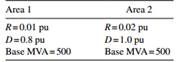### Create an Account

Home / Questions / Using the values of R and D in each area for Example 10B resolve for the 100MW load change...

# Using the values of R and D in each area for Example 10B resolve for the 100MW load change in area 1 under the following conditions Area 1 base MVA 2000 MVA Area 2 base MVA 500 MVA Then solve

Using the values of R and D in each area, for Example 10B, resolve for the 100-MW load change in area 1 under the following conditions:

Area 1: base MVA 2000 MVA

Area 2: base MVA 500 MVA

Then solve for a load change of 100 MW occurring in area 2 with R values and D values as in Example 10B and base MVA for each area as before.

Example 10B

You are given two system areas connected by a tie line with the following characteristics:Mar 29 2020 View more View LessSubscribe To Get Solution